# R k-means clustering and evaluation of the model

0
2168

The k-means clustering algorithms aim at partitioning n observations into a fixed number of k clusters. The algorithm will find homogeneous clusters. It works by dividing a large set of points (vectors) into groups having approximately the same number of points closest to them. Each group is represented by its centroid point. This type of clustering is an unsupervised learning technique. Clustering is mainly used for exploratory data mining.Convergence of k-means (https://en.wikipedia.org/wiki/K-means_clustering)

We can perform k-means clustering on a data matrix in R using the function “kmeans()”.

### Usage

kmeans(x, centers, iter.max = 10, nstart = 1, algorithm = c(“Hartigan-Wong”, “Lloyd”, “Forgy”, “MacQueen”), trace=FALSE)

# S3 method for kmeans

fitted(object, method = c(“centers”, “classes”), …)

### Dataset from clustring

```#We can genrate randome data:
x <- rbind(matrix(rnorm(100, sd = 0.3), ncol = 2),
matrix(rnorm(100, mean = 1, sd = 0.3), ncol = 2))
colnames(x) <- c("x", "y")
summary(x)
```
```##        x                  y
##  Min.   :-0.51232   Min.   :-0.4576
##  1st Qu.: 0.01864   1st Qu.: 0.0838
##  Median : 0.53680   Median : 0.4405
##  Mean   : 0.51812   Mean   : 0.5265
##  3rd Qu.: 1.03567   3rd Qu.: 1.0227
##  Max.   : 1.54161   Max.   : 1.8433
```
```#We also can use famous <i>Iris</i> flower data set.
summary(iris)
```
```##   Sepal.Length    Sepal.Width     Petal.Length    Petal.Width
##  Min.   :4.300   Min.   :2.000   Min.   :1.000   Min.   :0.100
##  1st Qu.:5.100   1st Qu.:2.800   1st Qu.:1.600   1st Qu.:0.300
##  Median :5.800   Median :3.000   Median :4.350   Median :1.300
##  Mean   :5.843   Mean   :3.057   Mean   :3.758   Mean   :1.199
##  3rd Qu.:6.400   3rd Qu.:3.300   3rd Qu.:5.100   3rd Qu.:1.800
##  Max.   :7.900   Max.   :4.400   Max.   :6.900   Max.   :2.500
##        Species
##  setosa    :50
##  versicolor:50
##  virginica :50
##
##
##
```

### Let’s starts with single variable (“single column at once”) clustering.

Sepal Length

```i <- grep("Sepal.Length", names(iris))
x <- iris[, i]
cl <- kmeans(x, 3, nstart = 100)
plot(x, col = cl\$cluster, main="Sepal.Length")
```Sepal Width

```i <- grep("Sepal.Width", names(iris))
x <- iris[, i]
cl <- kmeans(x, 3, nstart = 100)
plot(x, col = cl\$cluster, main="Sepal.Width")
```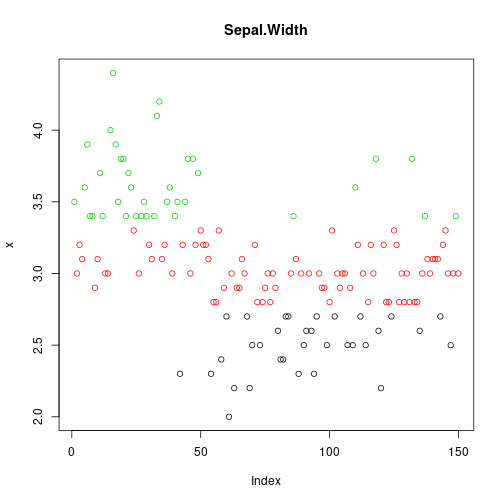Petal Length

```i <- grep("Petal.Length", names(iris))
x <- iris[, i]
cl <- kmeans(x, 3, nstart = 100)
plot(x, col = cl\$cluster, main="Petal.Length")
```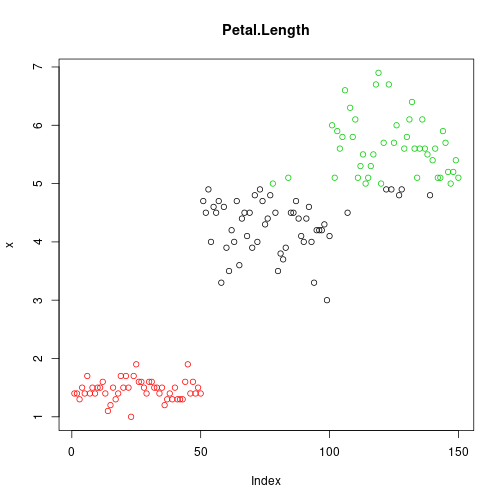Petal Width

```i <- grep("Petal.Width", names(iris))
x <- iris[, i]
cl <- kmeans(x, 3, nstart = 100)
plot(x, col = cl\$cluster, main="Petal.Width")
```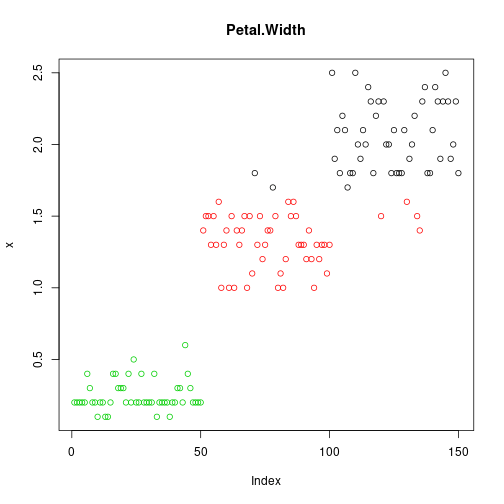Up to this point, it is clear that Petal feature is quite distinct comparing other plots. Now, Let’s try to cluster Sepal and Petal features combined.

Sepal

```i <- grep("Sepal", names(iris))
x <- iris[, i]
cl <- kmeans(x, 3, nstart = 100)
plot(x, col = cl\$cluster, main="Sepal")
```Petal

```i <- grep("Petal", names(iris))
x <- iris[, i]
cl <- kmeans(x, 3, nstart = 100)
plot(x, col = cl\$cluster, main="Petal")
```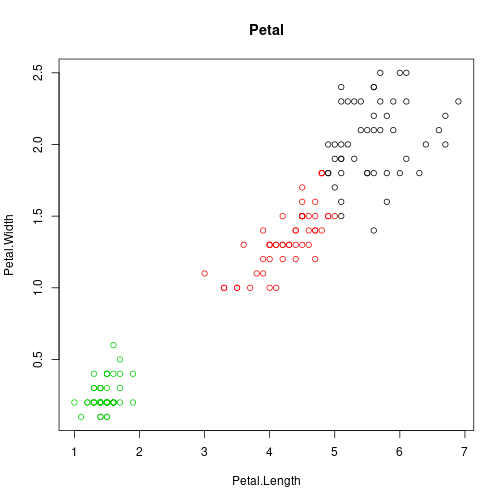Now, let’s do cluster using all four feature together.

Sepal and Petal

```i <- c(1,2,3,4)
x <- iris[, i]
cl <- kmeans(x, 3, nstart = 100)
plot(x, col = cl\$cluster, main="Sepal and Petal")
```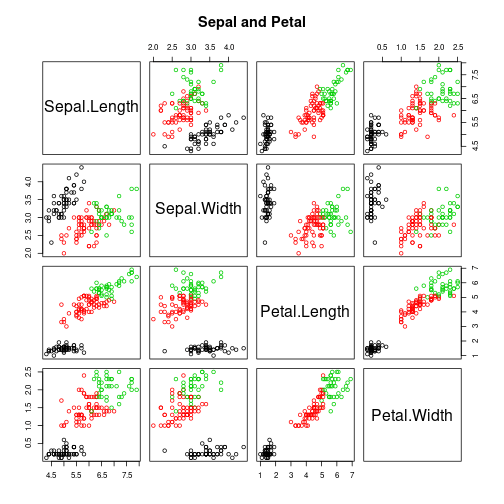Let’s check out the centers and size of each cluster.

```cl\$centers
```
```##   Sepal.Length Sepal.Width Petal.Length Petal.Width
## 1     5.006000    3.428000     1.462000    0.246000
## 2     5.901613    2.748387     4.393548    1.433871
## 3     6.850000    3.073684     5.742105    2.071053
```
```cl\$size
```
```##  50 62 38
```

### Evaluation of the model.

Finally, summarize our model.

```print(cl)
```
```## K-means clustering with 3 clusters of sizes 50, 62, 38
##
## Cluster means:
##   Sepal.Length Sepal.Width Petal.Length Petal.Width
## 1     5.006000    3.428000     1.462000    0.246000
## 2     5.901613    2.748387     4.393548    1.433871
## 3     6.850000    3.073684     5.742105    2.071053
##
## Clustering vector:
##    1 1 1 1 1 1 1 1 1 1 1 1 1 1 1 1 1 1 1 1 1 1 1 1 1 1 1 1 1 1 1 1 1 1 1
##   1 1 1 1 1 1 1 1 1 1 1 1 1 1 1 2 2 3 2 2 2 2 2 2 2 2 2 2 2 2 2 2 2 2 2
##   2 2 2 2 2 2 2 3 2 2 2 2 2 2 2 2 2 2 2 2 2 2 2 2 2 2 2 2 2 2 3 2 3 3 3
##  3 2 3 3 3 3 3 3 2 2 3 3 3 3 2 3 2 3 2 3 3 2 2 3 3 3 3 3 2 3 3 3 3 2 3
##  3 3 2 3 3 3 2 3 3 2
##
## Within cluster sum of squares by cluster:
##  15.15100 39.82097 23.87947
##  (between_SS / total_SS =  88.4 %)
##
## Available components:
##
##  "cluster"      "centers"      "totss"        "withinss"
##  "tot.withinss" "betweenss"    "size"         "iter"
##  "ifault"
```

A (between_SS / total_SS = 88.4 %) indicates good fit .

1.2.